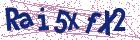# Nearest fuzzy number of type L-R to an arbitrary fuzzy number with applications to fuzzy linear system

Document Type : Research Article

Author

Department of Mathematics, Faculty of Basic Sciences, Arak Branch, Islamic Azad University, Arak, Iran.

Abstract

The fuzzy operations on fuzzy numbers of type L-R are much easier than general fuzzy numbers. It would be interesting to approximate a fuzzy number by a fuzzy number of type L-R. In this paper, we state and prove two significant application inequalities in the monotonic functions set. These inequalities show that under a condition, the nearest fuzzy number of type L-R to an arbitrary fuzzy number exists and is unique. After that, the nearest fuzzy number of type L-R can be obtained by solving a linear system. Note that the trapezoidal fuzzy numbers are a particular case of the fuzzy numbers of type L-R. The proposed method can represent the nearest trapezoidal fuzzy number to a given fuzzy number. Finally, to approximate fuzzy solutions of a fuzzy linear system, we apply our idea to construct a framework to find solutions of crisp linear systems instead of the fuzzy linear system. The crisp linear systems give the nearest fuzzy numbers of type L-R to fuzzy solutions of a fuzzy linear system. The proposed method is illustrated with some examples.

Keywords20.1001.1.24236977.2023.13.3.9.4

Main Subjects

#### References

 Abbasabandy, S. and Alavi, M. A method for solving fuzzy linear systems, Iran. J. Fuzzy Syst. 2(2) (2005) 37–43.
 Abbasbandy, S. and Amirfakhrian, M. The nearest trapezoidal form of a generalized left right fuzzy number, Internat. J. Approx. Reason. 43(2) (2006) 166–178.
 Abbasbandy, S.and Asady, B. A note on ‘‘A new approach for defuzzifi-cation’’[Fuzzy Sets and Systems 111 (2000), no. 3, 351–356; 1 748 552] by M. Ma, A. Kandel and M. Friedman, Fuzzy Sets Syst. 128 (2002) 131–132.
 Abbasbandy, S. and Asady, B. The nearest trapezoidal fuzzy number to a fuzzy quantity, Appl. Math. Comput. 156 (2004) 381–386.
 Abbasbandy, S. and Hajjari, T. Weighted trapezoidal approximation-preserving cores of a fuzzy number, Comput. Math. Appl. 59(9) (2010) 3066–3077.
 Amirfakhrian, M. A New Parametric Distance and Using it to Find The Nearest Approximation of A Fuzzy Number, Conference: 11th Iranian Conference of Fuzzy Systems, 2011.
 M. Amirfakhrian and S. Bagherian, The nearest approximation of a fuzzy number by a new parametric distance, Conference: 40th Annual Iranian Mathematics Conference, 2009.
 Ban, A.I and Coroianu, L. Nearest interval, triangular and trapezoidal approximation of a fuzzy number preserving ambiguity, Internat. J. Ap-prox. Reason. 53 (5) (2012) 805–836.
 Coroianu, L. Trapezoidal approximations of fuzzy numbers using quadratic programs,Fuzzy Sets Syst. 417 (2021) 71–92.
 Coroianu, L., Gagolewski, M. and Grzegorzewski, P. Piecewise linear approximation of fuzzy numbers: algorithms, arithmetic operations and stability of characteristics, Soft Comput. 23 (2019) 9491–9505.
 Dubois, D. and Prade, H. Fuzzy number: an overview, Fuzzy number: an overview,
 T. Hajjari, Approximation of fuzzy numbers via 0.5-Level and Mean core, Int. J. Appl. Math. Stat. (22) (2011) 69–78.
 T. Hajjari and H. Mahzoon, Approximation of a fuzzy number by two main characteristics, Mathematical Inverse Problems, 1(1) (2014) 101–111.
 Ghanbari, M., Allahviranloo, T., Nuraei, R. and Pedrycz, W. A new effective approximate multiplication operation on LR fuzzy numbers and its application, Soft Comput. 26(9)(2022) 4103–4113.
 Giachetti, R.E. and Young, R.E. A parametric representation of fuzzy numbers and their arithmetic operators, Fuzzy Sets Syst. 91 (2) (1997)185–202.
 Guerra, M.L. and Stefanini, L.Approximate fuzzy arithmetic operations using monotonic interpolations, Fuzzy Sets Syst. 150 (2005) 5–33.
 Klir, G.J., St. Clair, U. and Yuan, B., Fuzzy set theory: foundations and applications, Prentice-Hall Inc. 1997.
 Ma, M., Kandel, A. and Friedman, M. Correction to a new approach for the difizification, “[Fuzzy Sets and Systems 111 (2000) 351-356].” Fuzzy Sets Syst. 128 (1) (2002) 133–134.
 Zheng, Q. and Zhang, L. Global minimization of constrained problems with discontinuous penalty functions, Global optimization, control, and games, III, Comput. Math. Appl. 37 (1999), no. 4-5, 41–58.
 Voxman, W. Canonical representation of discrete fuzzy numbers, Fuzzy Set Syst. 118 (3) (2001) 557–466.
 Yeh, C.-T. Existence of interval, triangular, and trapezoidal approxima-tions of fuzzy numbers under a general condition, Fuzzy Sets Syst. 310 (2017) 1–13.
 Zadeh, L.A. The concept of a linguistic variable and its application to approximate reasoning-I, Inf. Sci. 8 (1975) 199–249.
 Zadeh L.A. The concept of a linguistic variable and its application to approximate reasoning-II, Inf. Sci. 8 (1975) , 301–357.
 Zadeh L.A. The concept of a linguistic variable and its application to approximate reasoning-III, Inf. Sci. 9 (1975) 43–80.
 Zhou, J., Yang, F. and Wang, K. Fuzzy arithmetic on LR fuzzy numbers with applications to fuzzy programming, J. Intell. Fuzzy Syst. 30 (2016) 71–87.